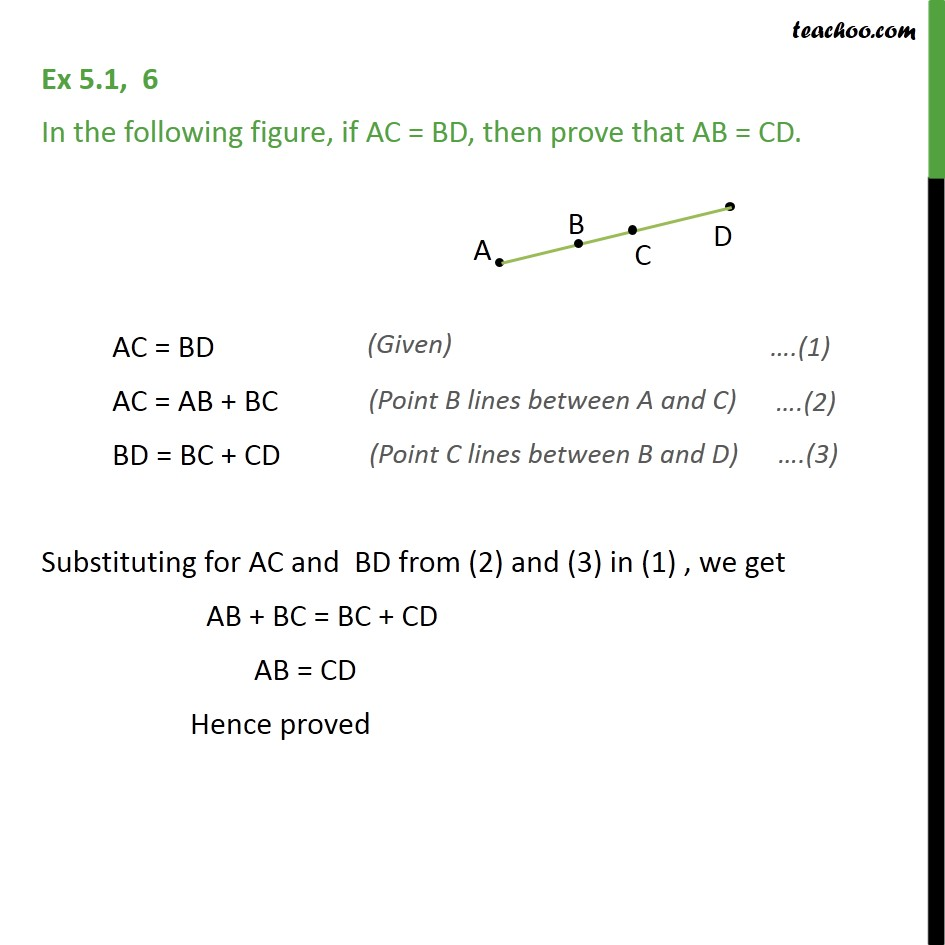1. Chapter 5 Class 9 Introduction to Euclid's Geometry (Deleted)
2. Serial order wise
3. Ex 5.1

Transcript

Ex 5.1, 6 In the following figure, if AC = BD, then prove that AB = CD. AC = BD AC = AB + BC BD = BC + CD Substituting for AC and BD from (2) and (3) in (1) , we get AB + BC = BC + CD AB = CD Hence proved

Ex 5.1

Chapter 5 Class 9 Introduction to Euclid's Geometry (Deleted)
Serial order wise